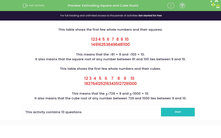# Estimate Square and Cube Roots

In this worksheet, students will estimate square roots and cube roots.Key stage:  KS 3

Curriculum topic:   Number

Curriculum subtopic:   Understand Integer Powers/Real Roots

Popular topics:   Estimation worksheets

Difficulty level:#### Worksheet Overview

This table shows the first few whole numbers and their squares.

 1 2 3 4 5 6 7 8 9 10 1 4 9 16 25 36 49 64 81 100

This means that the √81 = 9 and √100 = 10.

It also means that the square root of any number between 81 and 100 lies between 9 and 10.

This table shows the first few whole numbers and their cubes.

 1 2 3 4 5 6 7 8 9 10 1 8 27 64 125 216 343 512 729 1000

This means that the 3√729 = 9 and 3√1000 = 10.

It also means that the cube root of any number between 729 and 1000 lies between 9 and 10.

Let's have a go at some questions.

### What is EdPlace?

We're your National Curriculum aligned online education content provider helping each child succeed in English, maths and science from year 1 to GCSE. With an EdPlace account you’ll be able to track and measure progress, helping each child achieve their best. We build confidence and attainment by personalising each child’s learning at a level that suits them.

Get started